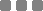## PROJECT:## PROJECT:

#### What lies behind usView Articles
Here is some sample text for an article. Here is some sample text for an article. Here is some sample text for an article. Here is some sample text for an article. Here is some sample text for an article. Here is some sample text for an article. Here is some sample text for an article. Here is some sample text for an article. Here is some sample text for an article. Here is some sample text for an article. Here is some sample text for an article. Here is some sample text for an article. Here is some sample text for an article. Here is some sample text for an article. Here is some sample text for an article. Here is some sample text for an article. Here is some sample text for an article. Here is some sample text for an article. Here is some sample text for an article. Here is some sample text for an article. Here is some sample text for an article.

Here is some sample text for an article. Here is some sample text for an article. Here is some sample text for an article. Here is some sample text for an article. Here is some sample text for an article. Here is some sample text for an article. Here is some sample text for an article. Here is some sample text for an article. Here is some sample text for an article. Here is some sample text for an article. Here is some sample text for an article. Here is some sample text for an article. Here is some sample text for an article. Here is some sample text for an article. Here is some sample text for an article. Here is some sample text for an article. Here is some sample text for an article. Here is some sample text for an article. Here is some sample text for an article. Here is some sample text for an article. Here is some sample text for an article.